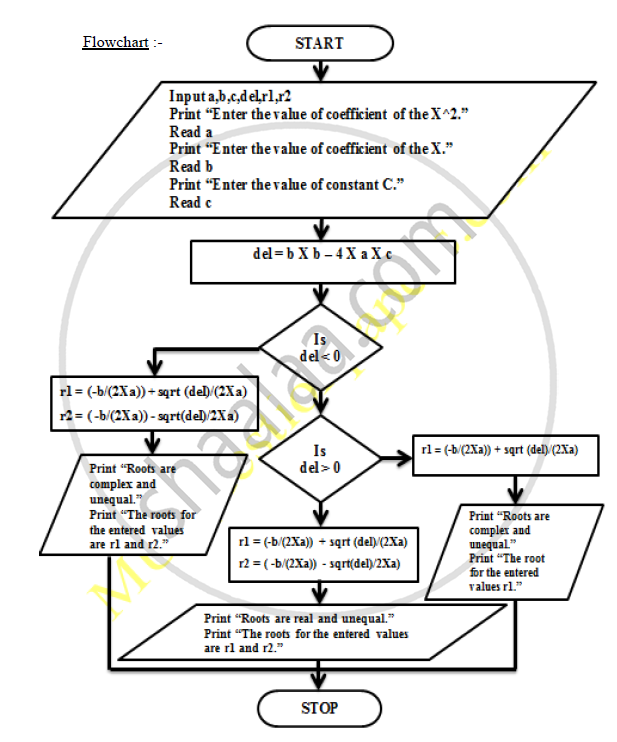# Draw the Flow Chart to Find Roots of a Quadratic Equation. - Structured Programming Approach

Draw the flow chart to find roots of a quadratic equation.

#### Solution

Ans.
Algorithm :-
Step I : Start.
Step II : Input a,b,c,del,r1,r2.
Step III : Print “Enter the value of coefficient of the X^2.”
Step IV : Read a.
Step V : Print “Enter the value of coefficient of the X.”
Step VI : Read b.
Step VII : Print “Enter the value of constant C.”
Step VIII : Read c.
Step IX : del = b X b – 4 X a X c
Step X : If del is less than zero Then,
r1 = ( -b / ( 2 X a ) ) + sqrt ( del ) / 2 X a
r2 = ( -b / ( 2 X a ) ) - sqrt ( del ) / 2 X a
Print “Roots are complex and unequal.”
Print “The roots for the entered values are r1                        and r2.”
Else - if del is greater than zero Then,
r1 = ( -b / ( 2 X a ) ) + sqrt ( del ) / 2 X a
r2 = ( -b / ( 2 X a ) ) - sqrt ( del ) / 2 X a
Print “Roots are real and unequal.”
Print “The roots for the entered values are r1                         and  r2.”
Else,
r1 = ( -b / ( 2 X a ) ) + sqrt ( del ) / 2 X a
Print “Roots are real and equal.”
Print “The root for the entered values is r1.”Concept: Three Construct of Algorithm and Flowchart
Is there an error in this question or solution?